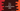# C++ program to print a multiplication table from 1 to n## C++ program to print a multiplication table:

In this post, we will learn how to write a C++ program to print a multiplication table. The multiplication table will be written for multiplication of the number with 1 to 10.

In our program, we will take the number to print the multiplication table as an input from the user and print the table to the user. The user can enter any number and the program will print the multiplication table.

With this program, you will learn how to read user inputs, how to use for loop and how to do mathematical calculations in C++.

### C++ program:

Below is the complete C++ program :

``````#include <iostream>
using namespace std;

int main()
{
int no;
cout << "Enter a number :" << endl;

cin >> no;
cout << "Multiplication table for " << no << " : " << endl;

for (int i = 1; i < 11; i++)
{
cout << no << " * " << i << " = " << no * i << endl;
}
}``````

### Explanation of this program:

Here,

• We are reading the number as user input using cin and storing that value in the variable no.
• The multiplication table is printed using a for loop.
• The for loop runs from i = 1 to i = 10. For each value of i, we are printing the multiplication table row for no * i.
• The cout in the for loop uses one endl at the end to add one newline.

### Sample Output:

``````Enter a number :
5
Multiplication table for 5 :
5 * 1 = 5
5 * 2 = 10
5 * 3 = 15
5 * 4 = 20
5 * 5 = 25
5 * 6 = 30
5 * 7 = 35
5 * 8 = 40
5 * 9 = 45
5 * 10 = 50

Enter a number :
10
Multiplication table for 10 :
10 * 1 = 10
10 * 2 = 20
10 * 3 = 30
10 * 4 = 40
10 * 5 = 50
10 * 6 = 60
10 * 7 = 70
10 * 8 = 80
10 * 9 = 90
10 * 10 = 100``````

### Multiplication tables from 1 to n:

We can also print all tables from 1 to n using two for loops and print them in a row. For example :

``````#include <iostream>
using namespace std;

int main()
{
int no;

cout << "Enter upper limit :" << endl;
cin >> no;

cout << "Multiplication table from 1 to " << no << " : " << endl;

for (int j = 1; j < no + 1; j++)
{
for (int i = 1; i < 11; i++)
{

cout << j << " * " << i << " = " << j * i << endl;
}
cout << endl
<< "------------" << endl
<< endl;
}
}``````

It will print the output as like below:

``````Enter upper limit :
4
Multiplication table from 1 to 4 :
1 * 1 = 1
1 * 2 = 2
1 * 3 = 3
1 * 4 = 4
1 * 5 = 5
1 * 6 = 6
1 * 7 = 7
1 * 8 = 8
1 * 9 = 9
1 * 10 = 10

------------

2 * 1 = 2
2 * 2 = 4
2 * 3 = 6
2 * 4 = 8
2 * 5 = 10
2 * 6 = 12
2 * 7 = 14
2 * 8 = 16
2 * 9 = 18
2 * 10 = 20

------------

3 * 1 = 3
3 * 2 = 6
3 * 3 = 9
3 * 4 = 12
3 * 5 = 15
3 * 6 = 18
3 * 7 = 21
3 * 8 = 24
3 * 9 = 27
3 * 10 = 30

------------

4 * 1 = 4
4 * 2 = 8
4 * 3 = 12
4 * 4 = 16
4 * 5 = 20
4 * 6 = 24
4 * 7 = 28
4 * 8 = 32
4 * 9 = 36
4 * 10 = 40

------------``````

Here, we are using the outer loop for each table and inner loop to print the content of each table. The outer loop runs from 1 to no.